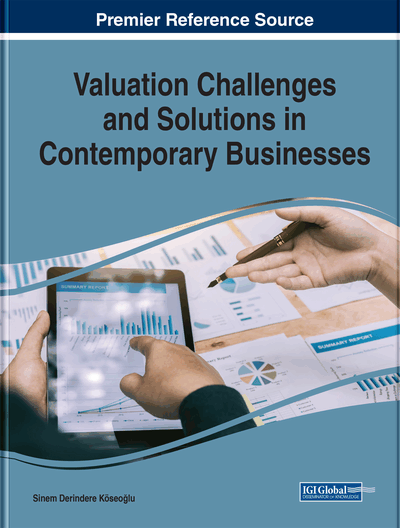# Challenges in Valuation by Using Discounted Free Cash Flow Method

Orhan Goker, Sinem Derindere Köseoğlu
DOI: 10.4018/978-1-7998-1086-5.ch004
OnDemand:
(Individual Chapters)
Available
\$37.50
No Current Special Offers

## Abstract

It is generally believed that, in determining the real value of a company, the best results are obtained by using the Dicounted FCF method. The overall value of the firm itself or the value of equity is determined by discounting the “appropriate” cash flows by “appropriate” discount rates. We basically need to determine three major parameters: free cash flows, cost of capital, and the terminal value. All these three parameters have sub-parameters within themselves. Because all these parameters and their sub-parameters are to be future values, many factors like the riskiness of the firm in question, its leverage ratio, whether it is a profitable firm, newly-established or public company will not only influence the calculation of these parameters/sub-parameters but will also make it more difficult for the analyst. This chapter explains what variables are needed for company valuation, how they are determined, and what problems may be faced in calculating these values. Finally, authors propose solutions to all the problems analysts will likely face.
Chapter Preview
Top

## Discounted Free Cash Flow (Fcf) Method

In this method, first the future cash flows are estimated and then discounted by the “appropriate rate of return’’ to find the firm or the equity value. Therefore, according to this method the value of a business or the value of the equity of the business is the present value of the expected cash flows over its expected life.

(1) where; E(FCF) represents expected free cash flow the firm will generate in the future, k; is required rate of return.

The full expression of the above formula is as shown below. If we discount the FCFF by WACC we will get the overall value of the firm, and if we discount the FCFE by the required rate of the shreholders (required rate of equity, cost of equity) we will get the value of equity of the firm. The FCFF or FCFE in the formula below are assumed to be constant.

(2)(3)
• ko=WACC (overall cost capital)

• ke=required rate of equity

Theoretically, the value of the firm determined by discounting the FCFF is equal to the value of the equity determined by discounting the FCFE plus the present value of the long-term debt.